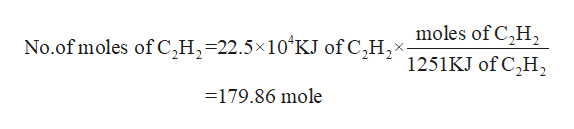# The flame in a torch used to cut metal is produced by burning acetylene (C2H2)(26.04 g/mol) in pure oxygen. Assuming the combustion of 1 mole of acetylene releases 1251 kJ of heat, what mass of acetylene is needed to cut through a piece of steel if the process requires 22.5  × 104 kJ of heat?                                            2 C2H2(g) + 5 O2(g) → 4 CO2(g) + 2 H2O(g) ΔH = –2502 kJ

Question
470 views

The flame in a torch used to cut metal is produced by burning acetylene (C2H2)(26.04 g/mol) in pure oxygen. Assuming the combustion of 1 mole of acetylene releases 1251 kJ of heat, what mass of acetylene is needed to cut through a piece of steel if the process requires 22.5  × 104 kJ of heat?                                            2 C2H2(g) + 5 O2(g) → 4 CO2(g) + 2 H2O(g) ΔH = –2502 kJ

check_circle

Step 1

Given that,

One mole of acetylene release heat = 1251 KJ

Molar mass of acetylene = 26.04g/mole

The reaction is

Step 2

Now, you calculate the no.of moles of acetylene for 22.5×104 KJ heat usinghelp_outlineImage Transcriptionclosemoles of C,H2 No.of moles of C,H-22.5x10 KJ of C,Hx 1251KJ of C2H2 -179.86 mole fullscreen
Step 3

Now, you calculate the m...

### Want to see the full answer?

See Solution

#### Want to see this answer and more?

Solutions are written by subject experts who are available 24/7. Questions are typically answered within 1 hour.*

See Solution
*Response times may vary by subject and question.
Tagged in

### Quantitative analysis